Recently, we published a new package dexterMML to the dexter github site, it will probably also be available from CRAN at some time in the future. What follows is the package vignette as it is at the time of writing.

In dexter we use an extended (polytomous) Rasch model to equate test forms and classical test analysis, distractor plots and the interaction model for quality control of items. For calibration of the NRM and interacton model we can use conditional maximum likelihood (CML).

CML calibration is not possible for fully adaptive tests or for Multi Stage tests with probabilistic routing rules. CML is currently also impractical for random tests (i.e. tests with items randomly selected from a bank). Classical analysis is also not feasible in these situations. So in these cases we must turn to Marginal Maximum Likelihood (MML) where we can use a marginal Rasch model (1PL) for equating and a 2PL for quality control of the items. As the name implies, in MML estimation the likelihood that is maximized is marginal, over an assumed distribution of ability. The likelihood for a response vector $$x$$ for a single person is defined as:

$P(x) = \int P(x|\theta, \omega)f(\theta|\phi,Y)d\theta$

with $$\theta$$ denoting ability and $$\omega$$ denoting a set of item parameters and $$Y$$ zero or more background variables. For unidimensional models, in practice $$f(\theta|\phi,Y)$$ is usually assumed to be a normal distribution or a combination of independent normal distributions with different parameters for each categorical value of $$Y$$. To identify the model, one or two population parameters can be fixed to an arbitrary value for a 1PL or a 2PL respectively, or the parameters of one or more items can be set to a fixed value. Here we want to focus on the background variable $$Y$$.

## Inclusion of background variables

To illustrate the effect of including background variables, we will simulate some data. We assume a population that consists of 3 different subgroups with a different distribution of the true ability $$\theta$$.

library(dexterMML)
library(dplyr)

population = data.frame(theta = c(rnorm(5000,-1,.5), rnorm(5000,0,1), rnorm(5000,1,2)),
Y = rep(letters[1:3],each=5000))

We take an item bank of 200 items from which we construct a fully random test for each subject.

nit = 200
items = data.frame(item_id = sprintf('item%03i',1:nit), item_score=1,
beta=runif(nit,-1,1), alpha=rnorm(nit,1,.2))

# simulate complete data
dat = sim_2pl(items, population$theta) # a random test of 30 items for(i in 1:nrow(dat)) dat[i,-sample(1:nit,30)] = NA Now we can proceed to estimate. f2 = fit_2pl(dat) check = inner_join(items, coef(f2), by=c('item_id','item_score'),suffix=c('.true','.est')) plot(check$alpha.true, check$alpha.est, xlab='true', ylab='estimated', main='alpha', bty='l', pch=20) plot(check$beta.true, check$beta.est, xlab='true', ylab='estimated', main='beta', bty='l', pch=20)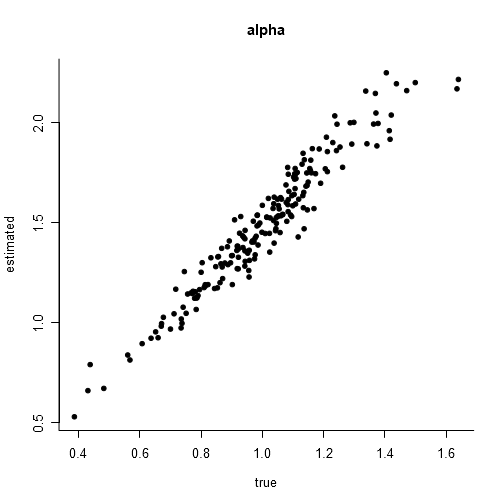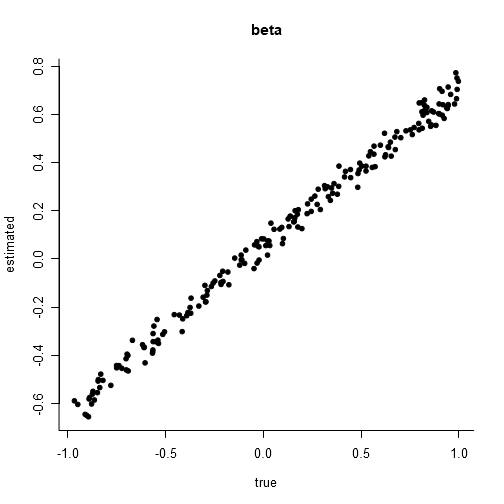Item parameters for a fully random test. True values are on the x-axis versus estimates on the y-axis. Ignoring an arbitrary identification constant, the estimates are reasonably close to the true values. However, at this point it can be noted that we severely misspecified the population distribution. The default assumption is a normal distribution, but because we used simulation we know that to be false. plot(density(population$theta), bty='l',xlab='', main='')

dn = function(mu,sigma) function(x) dnorm(x,mu,sigma)

plot(dn(mean(population$theta), sd(population$theta)),

for(y in unique(population$Y)) { pop = filter(population, Y==y) d = density(pop$theta)
d$y = d$y * nrow(pop)/nrow(population)
lines(d,col='gray')
}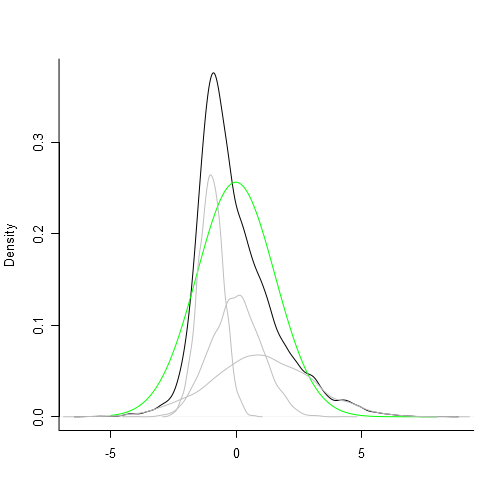Distribution of true ability (black line) and subgroups (gray lines). The green reference line shows the density of a normal distribution with the same mean and standard deviation as the true distribution.

Yet, even though we misspecified the population distribution, there is no visible bias in the item parameter estimates. Let’s try our random test again but this time we want to adapt our test a bit more to the individuals and so we use a targeted testing approach. Suppose that the item developers were able to provide an imperfect difficulty estimate for each item on a scale of 1 to 5. Further suppose that the background variable Y (schooltype) is known at the time of test administration, and from previous studies it is also known that the mean ability is highest in schooltype C and lowest in schooltype A. To enable a more precise measurement population A will get the easier items (difficulty 1-2), B the intermediate (2-4) and C the hardest items (4-5).

# an imperfect difficulty categorization
items = items %>%
mutate(df_cat = ntile(beta + runif(100,-.2,.2), 5),
j = row_number())

#simulate data
dat = sim_2pl(items, population$theta) # 3 overlapping item banks bank = list(a=filter(items, df_cat %in% 1:2)$j,
b=filter(items, df_cat %in% 2:4)$j, c=filter(items, df_cat %in% 4:5)$j)

# a targeted random test of 30 items
for(i in 1:nrow(dat))
dat[i, -sample(bank[[population$Y[i]]],30)] = NA If we calibrate on this new dataset, we see that omitting the relevant marginals leads to quite extreme bias in the item parameter estimates1. f2 = fit_2pl(dat) f2_marg = fit_2pl(dat, group=population$Y)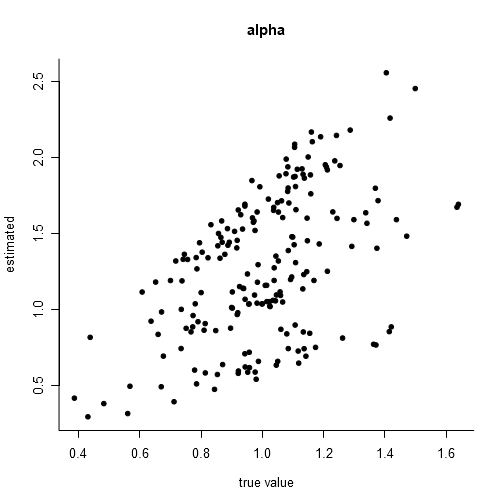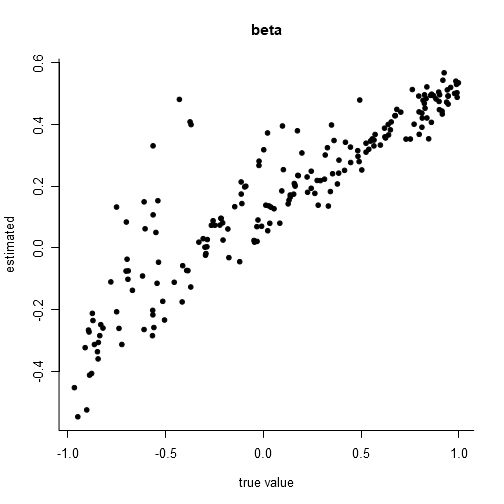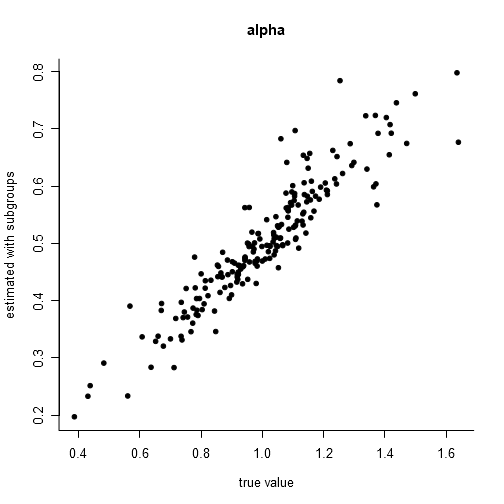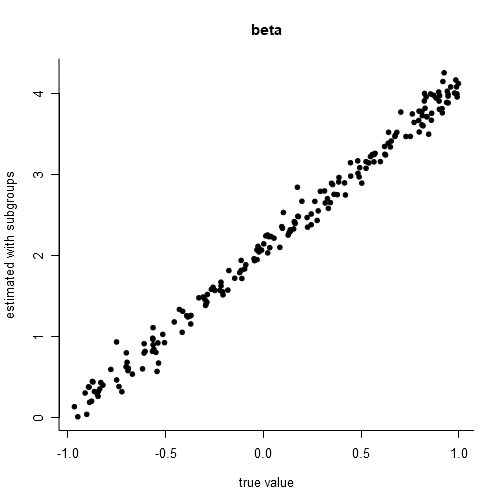Item parameters for a targeted random test. True values are on the x-axis versus estimates on the y-axis. The top two plots show the resulting parameters of a calibration in which the background variable Y is erroneously omitted, the bottom two plots show the results when the background variable is included.

It should be noted that in the first case, when we did not use the targeted test, we were strictly still wrong in omitting the background variable Y, since the different subgroups were not part of the same population. This is all perfectly explained in an excellent article by Eggen and Verhelst (2011)[Eggen, T. J. H. M., & Verhelst, N. D. (2011). The follwoing excerpt is from page 124. TTOP and TTMP stand for targeted testing in one or multiple (sub)populations respectively.

Summarizing we can say that in MML item calibration in complete testing designs is justified as long as we are sampling from one population there is more or less a free choice of whether the background variable is used in order to get estimates of the item parameters. However when sampling from multiple subpopulations and always in incomplete targeted testing designs, in TTOP as well as TTMP, there is no choice whether the background information Y must be used. Not using Y never leads to correct inferences on the item parameters or the population parameters. So we are obliged to use the subpopulation structure in MML estimation in order to get a correct estimation procedure. (…) Although standard computer implementation of MML procedures (…) have facilities to use Y, and to distinguish more subgroups in the samples, the awareness of the possible problems is not general and in practice many failures are made.

## Conclusion

In conclusion, whenever relevant background variables are known it is always safest to include them in an MML calibration, at least from a psychometric standpoint2. Whenever a targeted testing approach is used (i.e. a background variable influences the design), omission of the background variable will always lead to bias. This bias can range from the barely noticeable to the extreme, depending in a largely unknown and unverifiable manner on aspects of the design and the population distributions.

There are other ways of misspecifying a population distribution, e.g. the true population distribution might be skewed instead of Normal but, at least when estimating a 1PL or 2PL, in practice MML estimation is quite robust against this other type of misspecification so can be safely used.

1. The code for the plots is the same as before and is omitted here for brevity.↩︎

2. from a societal standpoint, this can be a wholly different matter↩︎